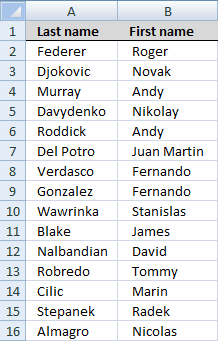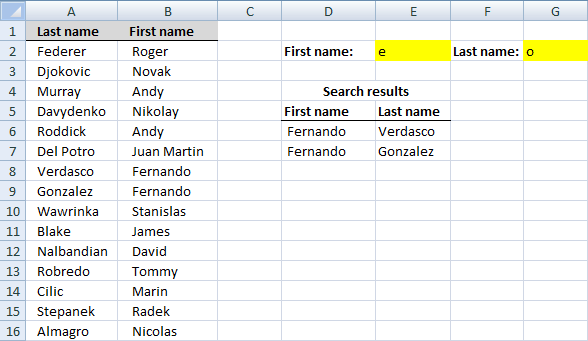Author: Oscar Cronquist Article last updated on December 27, 2018

### Question:

How do I search a list containing First name column and a last name column? I want to search both columns at the same time with two different criteria (one criteria for each column) and then display multiple search results.I created two search fields. First and last name in E2 and G2. The search results are presented in column D and E. See picture below.The array formula in cell D6:

=INDEX(\$B\$2:\$B\$16, SMALL(IF(ISNUMBER((SEARCH(\$E\$2, \$B\$2:\$B\$16))*(SEARCH(\$G\$2, \$A\$2:\$A\$16))), ROW(\$A\$2:\$A\$16)-MIN(ROW(\$A\$2:\$A\$16))+1, ""), ROW(A1)))

Recommended article

Search each column for a string each and return multiple records – OR logic

RU asks: Can you please suggest if i want to find out the rows with fixed value in "First Name" […]

### How to create an array formula

1. Copy (Ctrl + c) and paste (Ctrl + v) array formula into formula bar.
2. Press and hold Ctrl + Shift.
3. Press Enter once.
4. Release all keys.

How to enter an array formula

Array formulas allows you to do advanced calculations not possible with regular formulas.

Copy cell D6 and paste it down as far as needed.

The array formula in cell E6:

=INDEX(\$A\$2:\$A\$16, SMALL(IF(ISNUMBER((SEARCH(\$E\$2, \$B\$2:\$B\$16))*(SEARCH(\$G\$2, \$A\$2:\$A\$16))), ROW(\$A\$2:\$A\$16)-MIN(ROW(\$A\$2:\$A\$16))+1, ""), ROW(A1))) + CTRL + SHIFT + ENTER. Copy cell E6 and paste it down as far as needed.

Search for a text string in a data set and return multiple records

This article explains different techniques that filter rows/records that contain a given text string in any of the cell values […]

### Explaining the array formula in cell D6

Step 1 - Find rows where all criteria match

=INDEX(\$B\$2:\$B\$16, SMALL(IF(ISNUMBER((SEARCH(\$E\$2, \$B\$2:\$B\$16)*(SEARCH(\$G\$2, \$A\$2:\$A\$16))), ROW(\$A\$2:\$A\$16)-MIN(ROW(\$A\$2:\$A\$16))+1, ""), ROW(A1)))

Search() function returns the number of the character at which a specific character or text string is first found, reading left to right (not case sensitive).

SEARCH(\$E\$2, \$B\$2:\$B\$16)*(SEARCH(\$G\$2, \$A\$2:\$A\$16) returns this array:

(#VALUE, #VALUE, #VALUE, #VALUE, #VALUE, 24, 6,  #VALUE, #VALUE,#VALUE, #VALUE, #VALUE, #VALUE, #VALUE)

(SEARCH(\$E\$2, \$B\$2:\$B\$16)*(SEARCH(\$G\$2, \$A\$2:\$A\$16)) contains only absolute references.

How to use the SEARCH function

Returns a number representing the position a substring is, if found in a textstring. FIND is case sensitive and SEARCH is not.

Step 2 - Identify numbers in array

=INDEX(\$B\$2:\$B\$16, SMALL(IF(ISNUMBER((SEARCH(\$E\$2, \$B\$2:\$B\$16)*(SEARCH(\$G\$2, \$A\$2:\$A\$16))), ROW(\$A\$2:\$A\$16)-MIN(ROW(\$A\$2:\$A\$16))+1, ""), ROW(A1)))

ISNUMBER(value) checks whether a value is a number and returns TRUE or FALSE

ISNUMBER((SEARCH(\$E\$2, \$B\$2:\$B\$16)*(SEARCH(\$G\$2, \$A\$2:\$A\$16)))

becomes

ISNUMBER(#VALUE, #VALUE, #VALUE, #VALUE, #VALUE, 24, 6,  #VALUE, #VALUE,#VALUE, #VALUE, #VALUE, #VALUE, #VALUE))

and returns this array:

(FALSE, FALSE, FALSE, FALSE, FALSE, TRUE, TRUE, FALSE, FALSE, FALSE, FALSE, FALSE, FALSE, FALSE)

Step 3 - Convert boolean values into row numbers

=INDEX(\$B\$2:\$B\$16, SMALL(IF(ISNUMBER((SEARCH(\$E\$2, \$B\$2:\$B\$16)*(SEARCH(\$G\$2, \$A\$2:\$A\$16))), ROW(\$A\$2:\$A\$16)-MIN(ROW(\$A\$2:\$A\$16))+1, ""), ROW(A1)))

IF() checks whether a condition is met, and returns one value if TRUE, and another value if FALSE

IF(ISNUMBER((SEARCH(\$E\$2, \$B\$2:\$B\$16)*(SEARCH(\$G\$2, \$A\$2:\$A\$16))), ROW(\$A\$2:\$A\$16)-MIN(ROW(\$A\$2:\$A\$16))+1, "")

becomes

IF( (FALSE, FALSE, FALSE, FALSE, FALSE, TRUE, TRUE, FALSE, FALSE, FALSE, FALSE, FALSE, FALSE, FALSE) , ROW(\$A\$2:\$A\$16)-MIN(ROW(\$A\$2:\$A\$16))+1, "")

becomes

IF( (FALSE, FALSE, FALSE, FALSE, FALSE, TRUE, TRUE, FALSE, FALSE, FALSE, FALSE, FALSE, FALSE, FALSE) , (1,2,3,4,5,6,7,8,9, 10,11, 12,13, 14) , "")

and returns this array: ("","","","","", 6, 7, "", "", "", "", "", "", "")

How to use the IF function

Checks if a logical expression is met. Returns a specific value if TRUE and another specific value if FALSE.

Step 4 - Return k-th samllest value

=INDEX(\$B\$2:\$B\$16, SMALL(IF(ISNUMBER((SEARCH(\$E\$2, \$B\$2:\$B\$16)*(SEARCH(\$G\$2, \$A\$2:\$A\$16))), ROW(\$A\$2:\$A\$16)-MIN(ROW(\$A\$2:\$A\$16))+1, ""), ROW(A1)))

SMALL(array,k) returns the k-th smallest row number in this data set.

SMALL(IF(ISNUMBER((SEARCH(\$E\$2, \$B\$2:\$B\$16)*(SEARCH(\$G\$2, \$A\$2:\$A\$16))), ROW(\$A\$2:\$A\$16)-MIN(ROW(\$A\$2:\$A\$16))+1, ""), ROW(A1))

becomes

SMALL(("","","","","", 6, 7, "", "", "", "", "", "", ""), ROW(A1))

becomes

SMALL(("","","","","", 6, 7, "", "", "", "", "", "", ""), 1)

and returns 6

How to use the SMALL function

The SMALL function lets you extract a number in a cell range based on how small it is compared to the other numbers in the group.

Step 5 - Return a value of the cell at the intersection of a particular row and column

INDEX(array,row_num,[column_num])
Returns a value or reference of the cell at the intersection of a particular row and column, in a given range

=INDEX(\$B\$2:\$B\$16, SMALL(IF(ISNUMBER((SEARCH(\$E\$2, \$B\$2:\$B\$16))*(SEARCH(\$G\$2, \$A\$2:\$A\$16))), ROW(\$A\$2:\$A\$16)-MIN(ROW(\$A\$2:\$A\$16))+1, ""), ROW(A1)))

becomes

=INDEX(\$B\$2:\$B\$16,6) and returns Fernando in cell D6.

How to use the INDEX function

Gets a value in a specific cell range based on a row and column number.